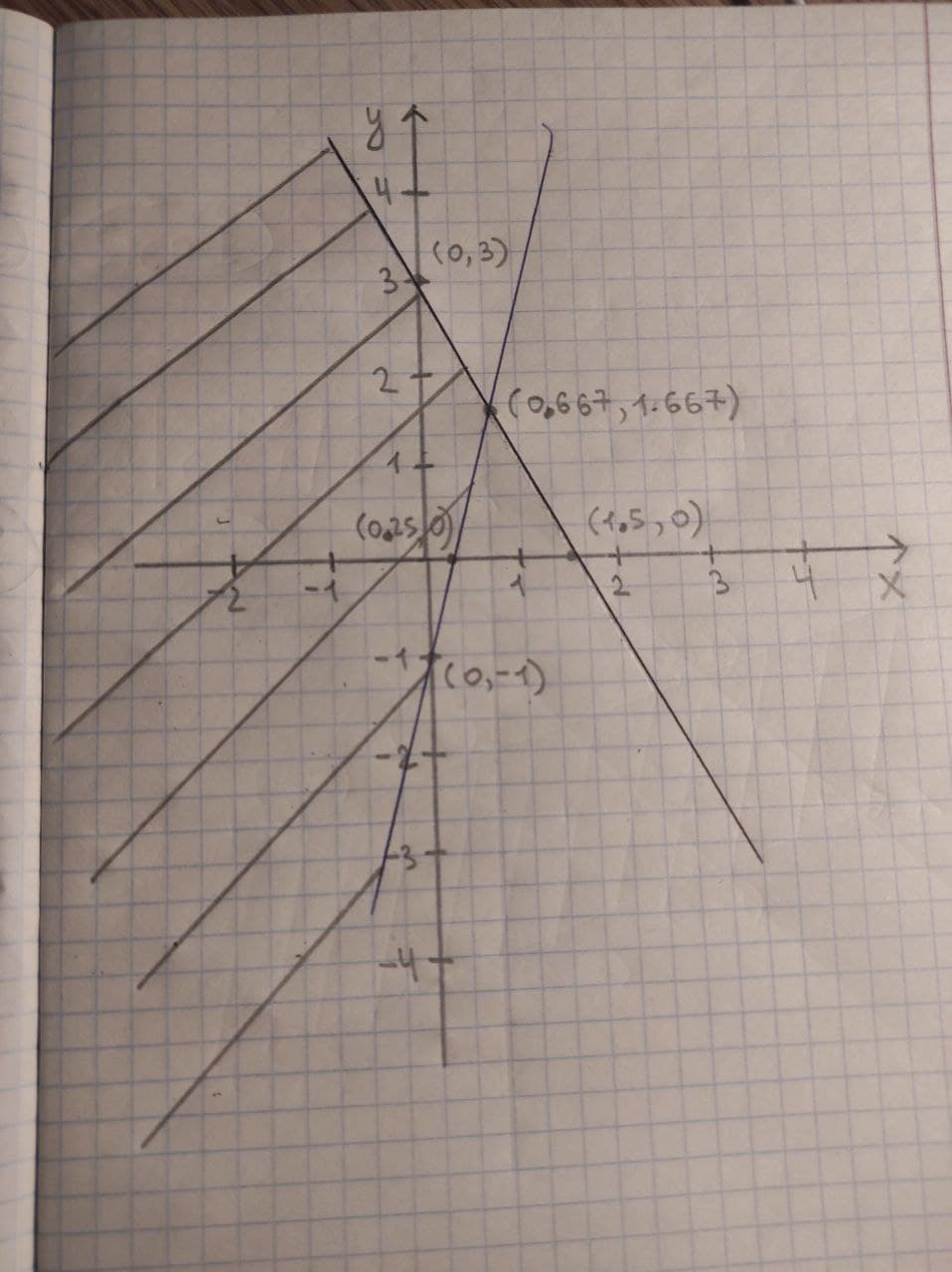# Graph the feasible region for the system of inequalities y>4x-1y<-2x+3DofotheroU 2021-03-07 Answered

Graph the feasible region for the system of inequalities
$y>4x-1$
$y←2x+3$

You can still ask an expert for help

## Want to know more about Inequalities systems and graphs?

• Questions are typically answered in as fast as 30 minutes

Solve your problem for the price of one coffee

• Math expert for every subject
• Pay only if we can solve itEzra Herbert
Step 1 Replace the inequality signs by equality signs and plot the two equations.
y>4x-1
y=4x-1 (1)
y<-2x+3
y=-2x+3 (2)
Now, check whether (0, 0) satisfies the inequalities.
If origin satisfies the inequality then the region containing origin is the region for inequality or vice-versa.
y>4x-1
For x=0:
4x-1=(4*0)-1=-1
As 0>-1, so y>4x-1 its true for (0,0).
Again, for x=0:
-2x+3=(-2*0)+3=3
As 0<3, so y<4x-1 is true for (0,0)
Step 2 Plot the region of inequality and the region satisfying both the inequalities is the feasible region. The shaded region is the feasible region.